In :
import pandas as pd
import matplotlib.pyplot as plt
import seaborn as sns
import numpy as np
import warnings
from datetime import datetime
import calendar
from math import sin, cos, sqrt, atan2, radians,asin
import folium
from folium import FeatureGroup, LayerControl, Map, Marker
from folium.plugins import HeatMap
from folium.plugins import TimestampedGeoJson
from folium.plugins import MarkerCluster
from geopy.distance import great_circle
import matplotlib.dates as mdates
import matplotlib as mpl
from datetime import timedelta
import datetime as dt
warnings.filterwarnings('ignore')
pd.set_option('display.max_colwidth', -1)
plt.style.use('fivethirtyeight')
import folium
from sklearn.cluster import KMeans
from sklearn import preprocessing
from sklearn.model_selection import train_test_split
from sklearn.preprocessing import Imputer
from sklearn import linear_model
from sklearn.metrics import mean_squared_error
from sklearn.ensemble import RandomForestRegressor
import pickle
from geopy.distance import geodesic

In :
##the training data has 55M rows. In this kernel, we shall only read in 6M rows

Out:
key fare_amount pickup_datetime pickup_longitude pickup_latitude dropoff_longitude dropoff_latitude passenger_count
0 2009-06-15 17:26:21.0000001 4.5 2009-06-15 17:26:21 UTC -73.844311 40.721319 -73.841610 40.712278 1
1 2010-01-05 16:52:16.0000002 16.9 2010-01-05 16:52:16 UTC -74.016048 40.711303 -73.979268 40.782004 1
2 2011-08-18 00:35:00.00000049 5.7 2011-08-18 00:35:00 UTC -73.982738 40.761270 -73.991242 40.750562 2
3 2012-04-21 04:30:42.0000001 7.7 2012-04-21 04:30:42 UTC -73.987130 40.733143 -73.991567 40.758092 1
4 2010-03-09 07:51:00.000000135 5.3 2010-03-09 07:51:00 UTC -73.968095 40.768008 -73.956655 40.783762 1
In :
train.info()

<class 'pandas.core.frame.DataFrame'>
RangeIndex: 6000000 entries, 0 to 5999999
Data columns (total 8 columns):
key                  object
fare_amount          float64
pickup_datetime      object
pickup_longitude     float64
pickup_latitude      float64
dropoff_longitude    float64
dropoff_latitude     float64
passenger_count      int64
dtypes: float64(5), int64(1), object(2)
memory usage: 366.2+ MB


### Convert pickup_datetime from Object to Datetime object¶

In :
train['pickup_datetime']=pd.to_datetime(train['pickup_datetime'],format='%Y-%m-%d %H:%M:%S UTC')

Out:
key fare_amount pickup_datetime pickup_longitude pickup_latitude dropoff_longitude dropoff_latitude passenger_count
0 2009-06-15 17:26:21.0000001 4.5 2009-06-15 17:26:21 -73.844311 40.721319 -73.841610 40.712278 1
1 2010-01-05 16:52:16.0000002 16.9 2010-01-05 16:52:16 -74.016048 40.711303 -73.979268 40.782004 1
2 2011-08-18 00:35:00.00000049 5.7 2011-08-18 00:35:00 -73.982738 40.761270 -73.991242 40.750562 2
3 2012-04-21 04:30:42.0000001 7.7 2012-04-21 04:30:42 -73.987130 40.733143 -73.991567 40.758092 1
4 2010-03-09 07:51:00.000000135 5.3 2010-03-09 07:51:00 -73.968095 40.768008 -73.956655 40.783762 1

### Create datetime features based on pickup_datetime¶

In :
train['pickup_date']= train['pickup_datetime'].dt.date
train['pickup_day']=train['pickup_datetime'].apply(lambda x:x.day)
train['pickup_hour']=train['pickup_datetime'].apply(lambda x:x.hour)
train['pickup_day_of_week']=train['pickup_datetime'].apply(lambda x:calendar.day_name[x.weekday()])
train['pickup_month']=train['pickup_datetime'].apply(lambda x:x.month)
train['pickup_year']=train['pickup_datetime'].apply(lambda x:x.year)


### Check for Missing Values¶

In :
train[pd.isnull(train)].sum()

Out:
key                   0.0
fare_amount           0.0
pickup_datetime       0.0
pickup_longitude      0.0
pickup_latitude       0.0
dropoff_longitude     0.0
dropoff_latitude      0.0
passenger_count       0.0
pickup_date           0.0
pickup_day            0.0
pickup_hour           0.0
pickup_day_of_week    0.0
pickup_month          0.0
pickup_year           0.0
dtype: float64

There are no missing values in the data.

### Exploratory Data Analysis¶

Distribution of Trip Fare

In :
plt.figure(figsize=(8,5))
sns.kdeplot(train['fare_amount']).set_title("Distribution of Trip Fare")

Out:
Text(0.5, 1.0, 'Distribution of Trip Fare')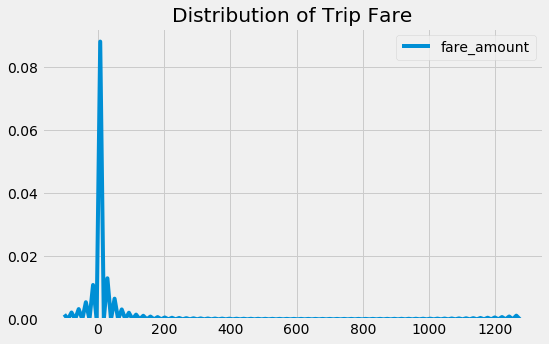There are some negative fare amount in the data and also it is skewed. Let us have a look at these data points

In :
train.loc[train['fare_amount']<0].shape

Out:
(262, 14)

There are 262 records with negative fare. We will remove these records from the data

In :
train=train.loc[train['fare_amount']>=0]
train.shape

Out:
(5999738, 14)

Since we saw above that fare amount is highly skewed,let us take log transformation of the fare amount and plot the distribution

In :
plt.figure(figsize=(8,5))
sns.kdeplot(np.log(train['fare_amount'].values)).set_title("Distribution of fare amount (log scale)")

Out:
Text(0.5, 1.0, 'Distribution of fare amount (log scale)')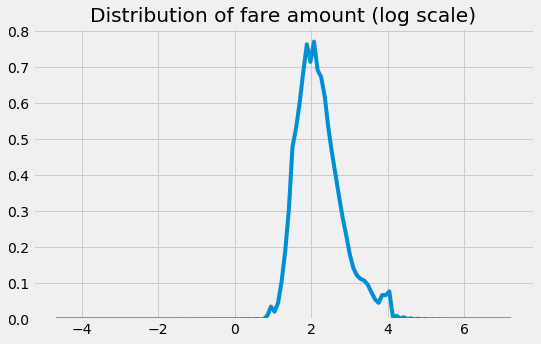1. Most fares are between 2.7 and 54 dollars .Median fare is around \$7

### Let us look at Geographical Features and their relationship with Fare Amount¶

Distribution of Pickup and Dropoff Lat Lng

In :
print("Range of Pickup Latitude is ", (min(train['pickup_latitude']),max(train['pickup_latitude'])))

Range of Pickup Latitude is  (-3488.079513, 3344.459268)

In :
print("Range of Dropoff Latitude is ", (min(train['dropoff_latitude']),max(train['dropoff_longitude'])))

Range of Dropoff Latitude is  (-3488.079513, 3457.62235)


The data is for Taxi Rides in Newyork, whose center lat, lng is at ((40,-74).The range of dropoff and pickup latitudes indicates lot of outlier locations in the train data.

In :
#Before we  ahead and identify outlier location, let us read the test data and see what the boundaries are.
print("Longitude Boundary in test data")
min(test.pickup_longitude.min(), test.dropoff_longitude.min()),max(test.pickup_longitude.max(), test.dropoff_longitude.max())

Longitude Boundary in test data

Out:
(-74.263242, -72.986532)
In :
print("Latitude Boundary in test data")
min(test.pickup_latitude.min(), test.pickup_latitude.min()),max(test.pickup_latitude.max(), test.pickup_latitude.max())

Latitude Boundary in test data

Out:
(40.573143, 41.709555)

Let us set the boundary for the train data also based on test data lat lng boundaries.We will mark the outlier locations as 1 and remove them for further analysi

In :
boundary={'min_lng':-74.263242,
'min_lat':40.573143,
'max_lng':-72.986532,
'max_lat':41.709555}


There are a lot of cases where lat lng is zero. How many such cases are there in the data?

In :
train[(train.pickup_latitude==0) | (train.pickup_longitude)==0 | (train.dropoff_latitude==0)|(train.dropoff_longitude==0)].shape

Out:
(114137, 14)

114K records have either pickup/dropoff lat/lng as 0.0 . There is a high chance this is present in test data as well. So, let us create a field called is_outlier_loc and mark as 1 in case it is an outlier. Any point beyond NYC border is an outlier. We will also drop all rows where the pickup or dropoff location is an outlier

In :
train.loc[~((train.pickup_longitude >= boundary['min_lng'] ) & (train.pickup_longitude <= boundary['max_lng']) &
(train.pickup_latitude >= boundary['min_lat']) & (train.pickup_latitude <= boundary['max_lat']) &
(train.dropoff_longitude >= boundary['min_lng']) & (train.dropoff_longitude <= boundary['max_lng']) &
(train.dropoff_latitude >=boundary['min_lat']) & (train.dropoff_latitude <= boundary['max_lat'])),'is_outlier_loc']=1
train.loc[((train.pickup_longitude >= boundary['min_lng'] ) & (train.pickup_longitude <= boundary['max_lng']) &
(train.pickup_latitude >= boundary['min_lat']) & (train.pickup_latitude <= boundary['max_lat']) &
(train.dropoff_longitude >= boundary['min_lng']) & (train.dropoff_longitude <= boundary['max_lng']) &
(train.dropoff_latitude >=boundary['min_lat']) & (train.dropoff_latitude <= boundary['max_lat'])),'is_outlier_loc']=0

print("Outlier vs Non Outlier Counts")
print(train['is_outlier_loc'].value_counts())

# Let us drop rows, where location is outlier
train=train.loc[train['is_outlier_loc']==0]
train.drop(['is_outlier_loc'],axis=1,inplace=True)

Outlier vs Non Outlier Counts
0.0    5871295
1.0    128443
Name: is_outlier_loc, dtype: int64


128K records are outliers

### Plot Heatmap of Pickups and Dropoffs within NYC¶

In :
city_long_border = (-74.03, -73.75)
city_lat_border = (40.63, 40.85)

train.plot(kind='scatter', x='dropoff_longitude', y='dropoff_latitude',
color='green',
s=.02, alpha=.6)
plt.title("Dropoffs")

plt.ylim(city_lat_border)
plt.xlim(city_long_border)

Out:
(-74.03, -73.75)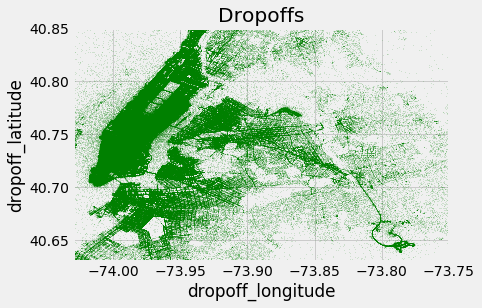In :
train.plot(kind='scatter', x='pickup_longitude', y='pickup_latitude',
color='blue',
s=.02, alpha=.6)
plt.title("Pickups")

plt.ylim(city_lat_border)
plt.xlim(city_long_border)

Out:
(-74.03, -73.75)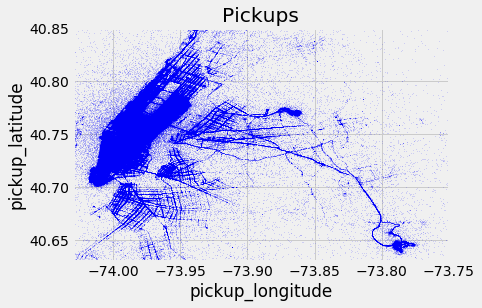Apart from Manhattan, we can see heavy pickups and dropoffs near JFK and La Guardia Airport.

Heatmap based on fare amount across NYC

In :
# Let us round pickup and dropoff lat lng to 3 decimal places
train['pickup_latitude_round3']=train['pickup_latitude'].apply(lambda x:round(x,3))
train['pickup_longitude_round3']=train['pickup_longitude'].apply(lambda x:round(x,3))
train['dropoff_latitude_round3']=train['dropoff_latitude'].apply(lambda x:round(x,3))
train['dropoff_longitude_round3']=train['dropoff_longitude'].apply(lambda x:round(x,3))

In :
pickup_fare_amount=train.groupby(['pickup_latitude_round3','pickup_longitude_round3'])['fare_amount'].mean().reset_index().rename(columns={'fare_amount':'avg_fare'})

Out:
pickup_latitude_round3 pickup_longitude_round3 avg_fare
0 40.573 -74.228 8.5
1 40.573 -73.983 13.5
2 40.574 -74.243 5.7
3 40.574 -74.224 4.1
4 40.574 -74.223 4.5

## In the scatter plot, we saw the high density of pickups and dropoffs from and to JFK and La Guardia Airport¶

Let us look at over time how fares are from La Guardia and JFK

In :
JFK={'min_lng':-73.8352,
'min_lat':40.6195,
'max_lng':-73.7401,
'max_lat':40.6659}
JFK_center=[40.6437,-73.7900]
# Get all pickups to JFK
JFK_data=train.loc[(train.pickup_latitude>=JFK['min_lat']) & (train.pickup_latitude<=JFK['max_lat'])]
JFK_data=JFK_data.loc[(train.pickup_longitude>=JFK['min_lng']) & (train.pickup_longitude<=JFK['max_lng'])]

print("Number of Trips with Pickups from JFK",JFK_data.shape)

JFK_dropoff=train.loc[(train.dropoff_latitude>=JFK['min_lat']) & (train.dropoff_latitude<=JFK['max_lat'])]
JFK_dropoff=JFK_dropoff.loc[(train.dropoff_longitude>=JFK['min_lng']) & (train.dropoff_longitude<=JFK['max_lng'])]

print("Number of Trips with Dropoffs to JFK",JFK_dropoff.shape)

'''
# Create a folium map with JFK as the center
m=folium.Map(location =JFK_center,zoom_start = 10,)

#Add markers in blue for each pickup location and line between JFK and Pickup location over time. The thickness of line indicates the fare_amount

for index,row in JFK_data.iterrows():
'''


Number of Trips with Pickups from JFK 90182
Number of Trips with Dropoffs to JFK 45609

Out:
"\n# Create a folium map with JFK as the center \nm=folium.Map(location =JFK_center,zoom_start = 10,)\nfolium.Marker(location=JFK_center, popup='JFK Airport',icon=folium.Icon(color='black')).add_to(m)\n\n#mc = MarkerCluster().add_to(m)\n#Add markers in blue for each pickup location and line between JFK and Pickup location over time. The thickness of line indicates the fare_amount\n\nfor index,row in JFK_data.iterrows():\n    folium.Marker([row['dropoff_latitude'],row['dropoff_longitude']]).add_to(m)\n"

What is the Average Fare amount of trips from JFK

In :
plt.figure(figsize=(8,5))
sns.kdeplot(np.log(JFK_data['fare_amount'].values),label='JFK Pickups')
#sns.kdeplot(np.log(JFK_dropoff['fare_amount'].values),label='JFK Dropoff')
sns.kdeplot(np.log(train['fare_amount'].values),label='All Trips in Train data')
plt.title("Fare Amount Distribution")

Out:
Text(0.5, 1.0, 'Fare Amount Distribution')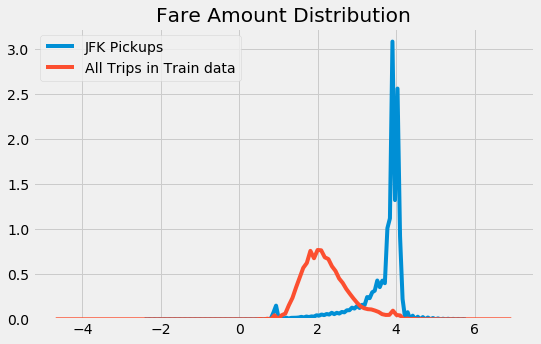As we can see, the fare amount is much higher when pickup is from JFK.

In :
plt.figure(figsize=(8,5))
sns.kdeplot(np.log(JFK_dropoff['fare_amount'].values),label='JFK')
sns.kdeplot(np.log(train['fare_amount'].values),label='train')
plt.title("Dropoffs vs Fare Amount")

Out:
Text(0.5, 1.0, 'Dropoffs vs Fare Amount')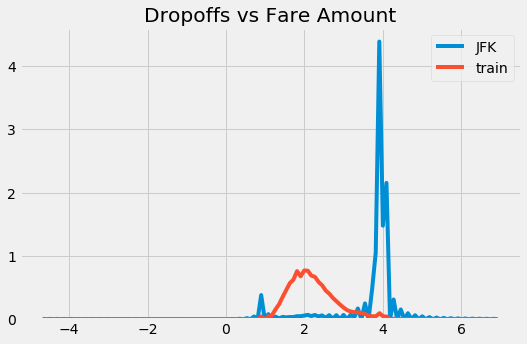In :
del JFK_data
del JFK
del JFK_dropoff


Distribution of fare amount for both pickup and dropoff to JFK is similar

In :
## Based on the above, let us create a function to see whether pickup or dropoff is an Airport.

'''
def isAirport(latitude,longitude,airport_name='JFK'):
if airport_name=='JFK':
boundary={'min_lng':-73.8352,
'min_lat':40.6195,
'max_lng':-73.7401,
'max_lat':40.6659}
elif airport_name=='EWR':
boundary={
'min_lng':-74.1925,
'min_lat':40.6700,
'max_lng':-74.1531,
'max_lat':40.7081

}
elif airport_name=='la guardia':
boundary={'min_lng':-73.8895,
'min_lat':40.7664,
'max_lng':-73.8550,
'max_lat':40.7931
}
if latitude>=boundary['min_lat'] and latitude<=boundary['max_lat']:
if longitude>=boundary['min_lng'] and longitude<=boundary['max_lng']:
return 1
else:
return 0

'''

nyc_airports={'JFK':{'min_lng':-73.8352,
'min_lat':40.6195,
'max_lng':-73.7401,
'max_lat':40.6659},

'EWR':{'min_lng':-74.1925,
'min_lat':40.6700,
'max_lng':-74.1531,
'max_lat':40.7081

},
'LaGuardia':{'min_lng':-73.8895,
'min_lat':40.7664,
'max_lng':-73.8550,
'max_lat':40.7931

}

}
def isAirport(latitude,longitude,airport_name='JFK'):

if latitude>=nyc_airports[airport_name]['min_lat'] and latitude<=nyc_airports[airport_name]['max_lat'] and longitude>=nyc_airports[airport_name]['min_lng'] and longitude<=nyc_airports[airport_name]['max_lng']:
return 1
else:
return 0

In :
train['is_pickup_JFK']=train.apply(lambda row:isAirport(row['pickup_latitude'],row['pickup_longitude'],'JFK'),axis=1)
train['is_dropoff_JFK']=train.apply(lambda row:isAirport(row['dropoff_latitude'],row['dropoff_longitude'],'JFK'),axis=1)

In :
train['is_pickup_EWR']=train.apply(lambda row:isAirport(row['pickup_latitude'],row['pickup_longitude'],'EWR'),axis=1)
train['is_dropoff_EWR']=train.apply(lambda row:isAirport(row['dropoff_latitude'],row['dropoff_longitude'],'EWR'),axis=1)

In :
train['is_pickup_la_guardia']=train.apply(lambda row:isAirport(row['pickup_latitude'],row['pickup_longitude'],'LaGuardia'),axis=1)
train['is_dropoff_la_guardia']=train.apply(lambda row:isAirport(row['dropoff_latitude'],row['dropoff_longitude'],'LaGuardia'),axis=1)


Trip Distance

In :
#calculate trip distance in miles
def distance(lat1, lat2, lon1,lon2):
p = 0.017453292519943295 # Pi/180
a = 0.5 - np.cos((lat2 - lat1) * p)/2 + np.cos(lat1 * p) * np.cos(lat2 * p) * (1 - np.cos((lon2 - lon1) * p)) / 2
return 0.6213712 * 12742 * np.arcsin(np.sqrt(a))

In :
train['trip_distance']=train.apply(lambda row:distance(row['pickup_latitude'],row['dropoff_latitude'],row['pickup_longitude'],row['dropoff_longitude']),axis=1)

In :
sns.kdeplot(np.log(train['trip_distance'].values)).set_title("Distribution of Trip Distance (log scale)")

Out:
Text(0.5, 1.0, 'Distribution of Trip Distance (log scale)')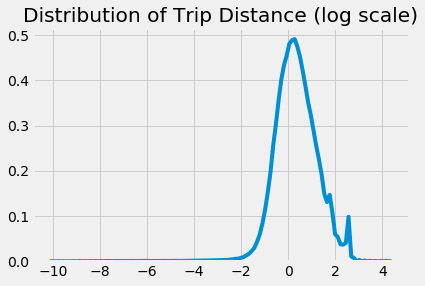In :
plt.scatter(x=train['trip_distance'],y=train['fare_amount'])
plt.xlabel("Trip Distance")
plt.ylabel("Fare Amount")

Out:
Text(0, 0.5, 'Fare Amount')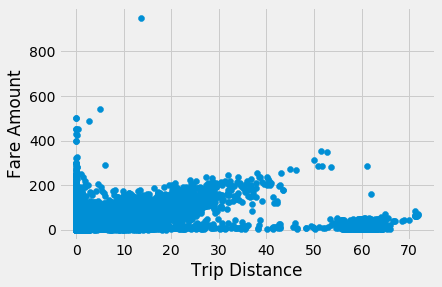The fare seems to be fixed for trip distances > 50 miles. Normally airports pickup or dropoff have fixed prices. We can remove the airport pickup and dropoff and plot the distribution of Fare Amount vs Trip distribution

In :
non_airport=train.loc[(train['is_dropoff_JFK']==0) & (train['is_dropoff_EWR']==0) & (train['is_dropoff_la_guardia']==0)]
non_airport=non_airport.loc[(non_airport['is_pickup_JFK']==0) & (non_airport['is_pickup_EWR']==0) & (non_airport['is_pickup_la_guardia']==0)]
non_airport.shape

Out:
(5547254, 25)
In :
plt.scatter(x=non_airport['trip_distance'],y=non_airport['fare_amount'])
plt.xlabel("Trip Distance")
plt.ylabel("Fare Amount")
plt.title("Trip Distance vs Fare Amount (excluding airport rides)")

Out:
Text(0.5, 1.0, 'Trip Distance vs Fare Amount (excluding airport rides)')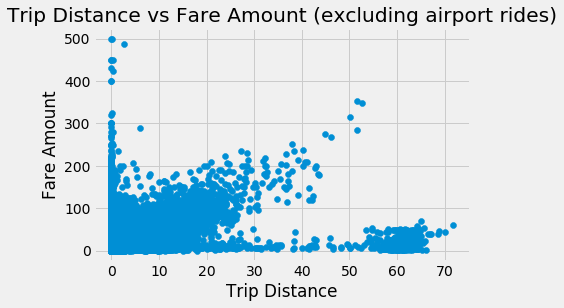In the plot above, we can see two clusters with linear realtionship between taxi fare and distance. But for trip distances >50miles, though a linear relationship exists,the fare amount is very low. Let us check where these trips originate and end

In :
non_airport_long_trips=non_airport[non_airport['trip_distance']>=50]

In :
drop_map = folium.Map(location = [40.730610,-73.935242],zoom_start = 12,)
#print(pickup.shape)
### For each pickup point add a circlemarker

for index, row in non_airport_long_trips.iterrows():

folium.CircleMarker([row['dropoff_latitude_round3'], row['dropoff_longitude_round3']],

color="#008000",
fill_opacity=0.9
for index, row in non_airport_long_trips.iterrows():

folium.CircleMarker([row['pickup_latitude_round3'], row['pickup_longitude_round3']],

color="blue",
fill_opacity=0.9

'''
hm_wide = HeatMap( list(zip(drop.dropoff_latitude_round3.values, drop.dropoff_longitude_round3.values, drop.Num_Trips.values)),
min_opacity=0.2,